# Q2 a) Find the second derivative of the following function y = tan x + sin-1x...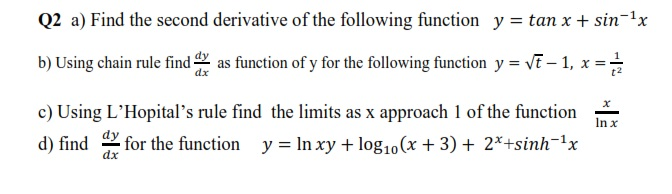Q2 a) Find the second derivative of the following function y = tan x + sin-1x b) Using chain rule find any as function of y for the following function y = Vē – 1, x = t2 In x c) Using L'Hopital's rule find the limits as x approach 1 of the function d) find for the function y = ln xy + log10 (x + 3) + 2*+sinh-1x dx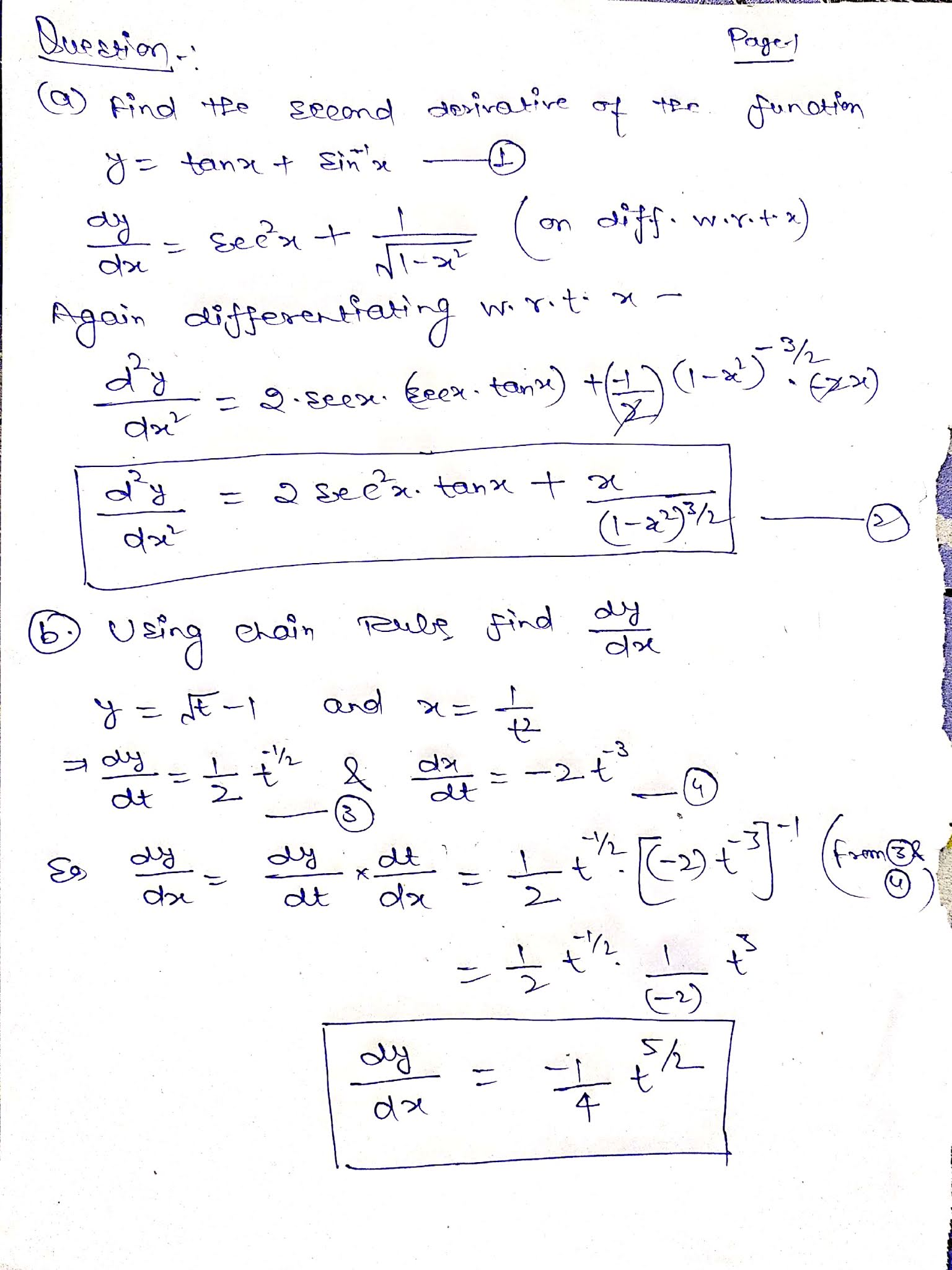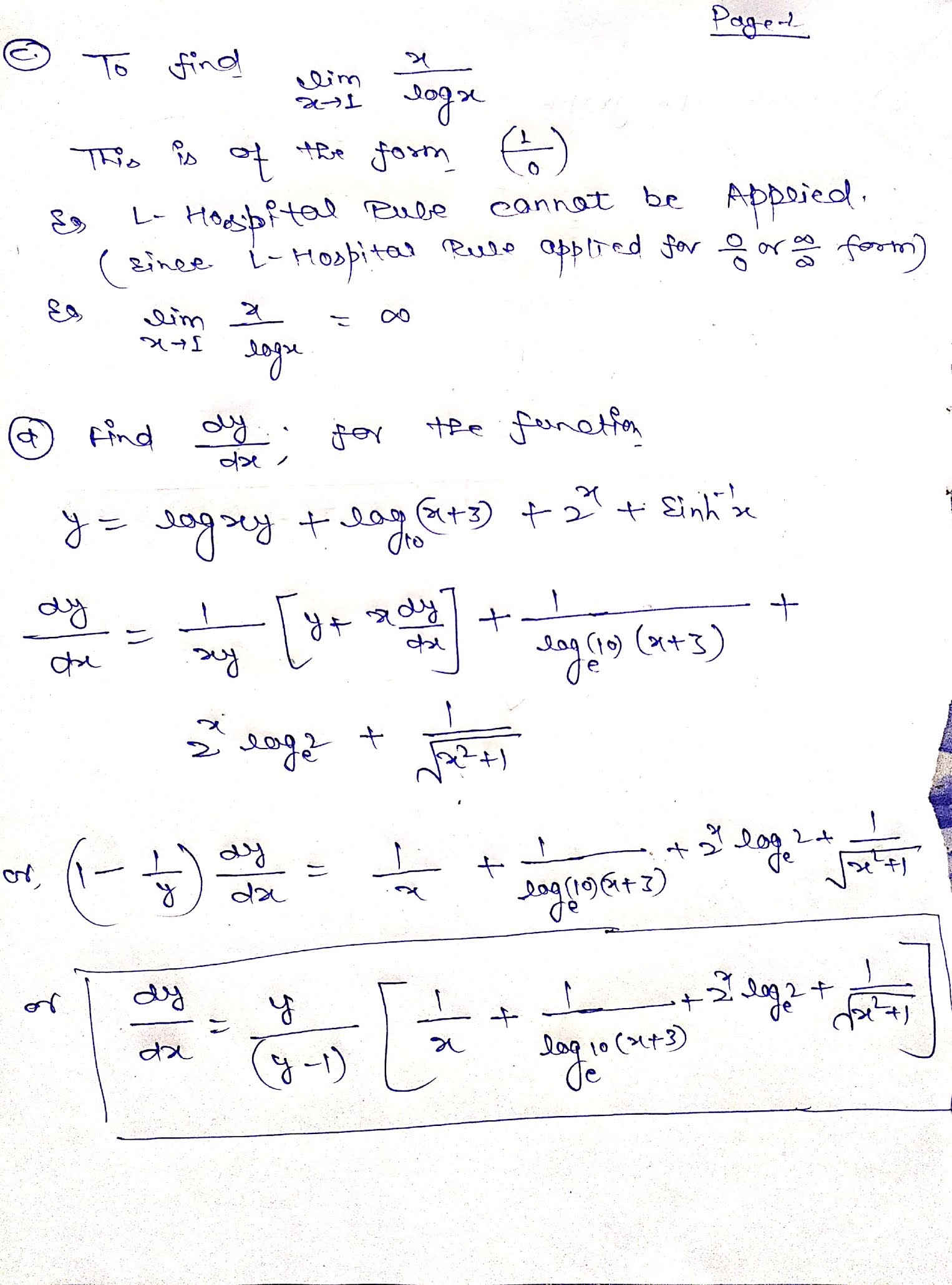##### Add Answer of: Q2 a) Find the second derivative of the following function y = tan x + sin-1x...
Similar Homework Help Questions
• ### please do #7 the derivative of the function y - tan-(x-v1+x? ). Problem 5. Find the...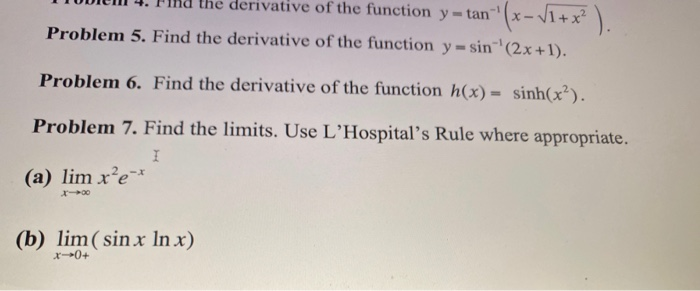please do #7 the derivative of the function y - tan-(x-v1+x? ). Problem 5. Find the derivative of the function y = sin(2x+1). Problem 6. Find the derivative of the function h(x) = sinh(x?). Problem 7. Find the limits. Use L'Hospital's Rule where appropriate. I (a) lim x’e-* (b) lim (sin x In x) x0+

• ### Find the derivative of the function. y sin-1(5x+ 1) Part 1 of 3 The function y - sin-1(5x + 1) is a composition, and...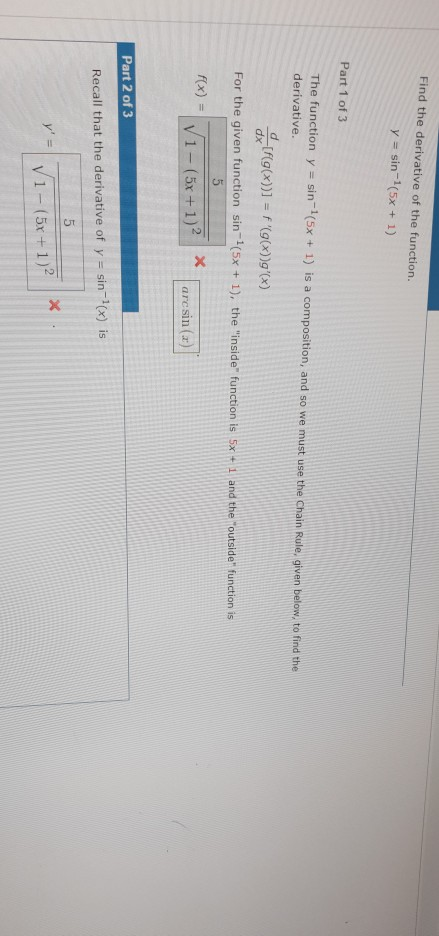Find the derivative of the function. y sin-1(5x+ 1) Part 1 of 3 The function y - sin-1(5x + 1) is a composition, and so we must use the Chain Rule, given below, to find the derivative dx[f(g(x))) = f '(g(x))g'(x) For the given function sin 1(5x+ 1), the "inside" function is Sx + and the foutside" function is arcsin (a) Part 2 of 3 Recall that the derivative of y sin-1(x) is 1-(5x - 1)2 Find the derivative of...

• ### Q2) Find the derivative of each function a) f(1) = b) f(x) sin 1COSI 1+008 d)...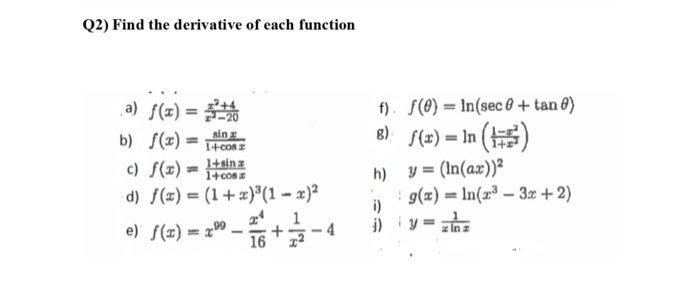Q2) Find the derivative of each function a) f(1) = b) f(x) sin 1COSI 1+008 d) f(x) = (1 + x)'(1 - x)2 1 e) f(1) = 2009 1672 f). f() = ln(sec 0 + tan ) B): S(21) = 1n () h) y = (In(ax)? g(x) = ln(2.3 - 3x + 2) i) c) f(x) = sina

• ### Using the Chain rule find the derivative of the function: 1. y=(x?+2x2,6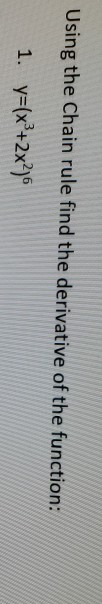Using the Chain rule find the derivative of the function: 1. y=(x?+2x2,6

• ### Find the directions in which the function increases and decreases most rapidly at Po. Then find the derivatives of the function in these directions. xy) =x"cos(y) +x"win(x) cos(x)sin(y). Plo...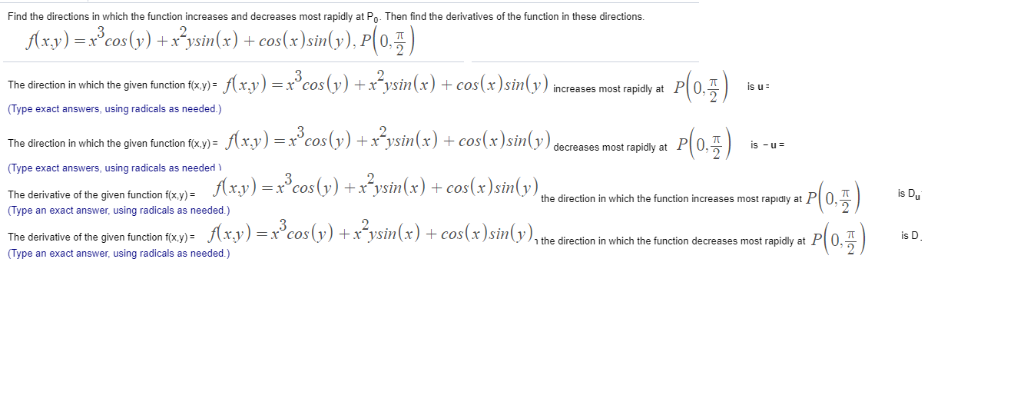Find the directions in which the function increases and decreases most rapidly at Po. Then find the derivatives of the function in these directions. xy) =x"cos(y) +x"win(x) cos(x)sin(y). Plo The direction in which the given function txy_f(xy)-x3cos(v)+x2vsin(x) + cos(x)sin(y)increases most rapidly at P 0주 is u: " (Type exact answers, using radicals as n (xy)=x3cos(y)+x"win(x)-cos(x)sin(y) The direc on in which the given function f(xy- is eases most rapidly at (Type exact answers, using radicals as needed The derivative of the...

• ### If y squre =sin x/(1+ cos x ) proof that second derivative of y =y(1- cos 2x)/(4 sin square x) ?

If #y^2 =sin x/(1+ cos x )# prove that second derivative of y#=(d^2y)/(dx^2) =(y(1-2 cosx))/(4 sin^2 x)# ?

• ### Problem 1. (a) The radius of a sphere is increasing at a rate of 4 mm/s....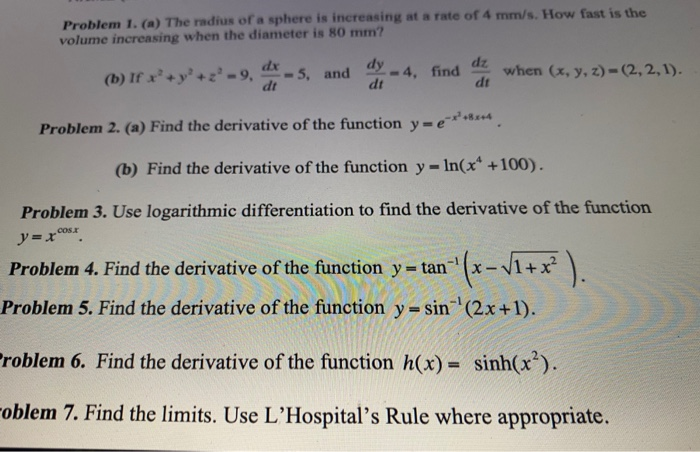Problem 1. (a) The radius of a sphere is increasing at a rate of 4 mm/s. How fast is the volume increasing when the diameter is 80 mm? dy dx (b) If x+y+z-9, dt 5, and -4, find dt dz dt when (x, y, z)-(2,2,1). Problem 2. (a) Find the derivative of the function yr e-****** (b) Find the derivative of the function y - In(x* +100). cos. Problem 3. Use logarithmic differentiation to find the derivative of the function...

• ### 11. (5 points each) Find the derivative of each function. DO NOT simplify your answers. cos(x)...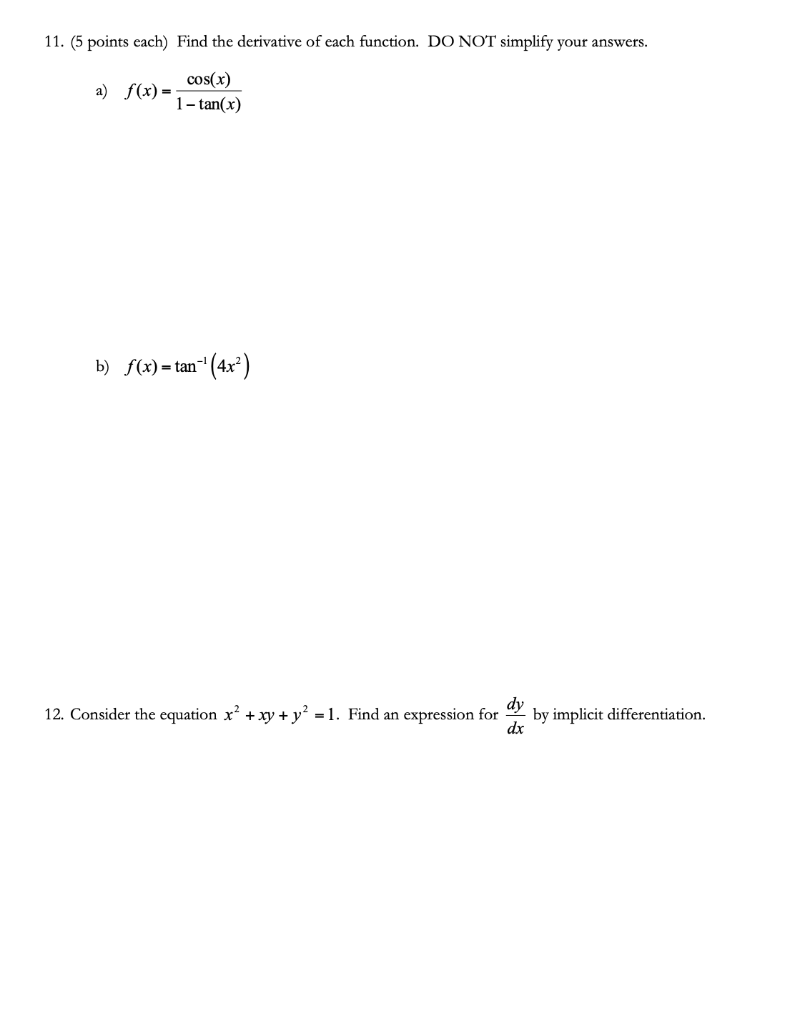11. (5 points each) Find the derivative of each function. DO NOT simplify your answers. cos(x) a) f(x) = 1-tan(x) b) f(x) – tan-" (4x²) dy 12. Consider the equation x² + xy + y² = 1. Find an expression for by implicit differentiation. dx

• ### r- 3X -4 1-Given the function: y=-- decide if f(x) = y is continuous or has...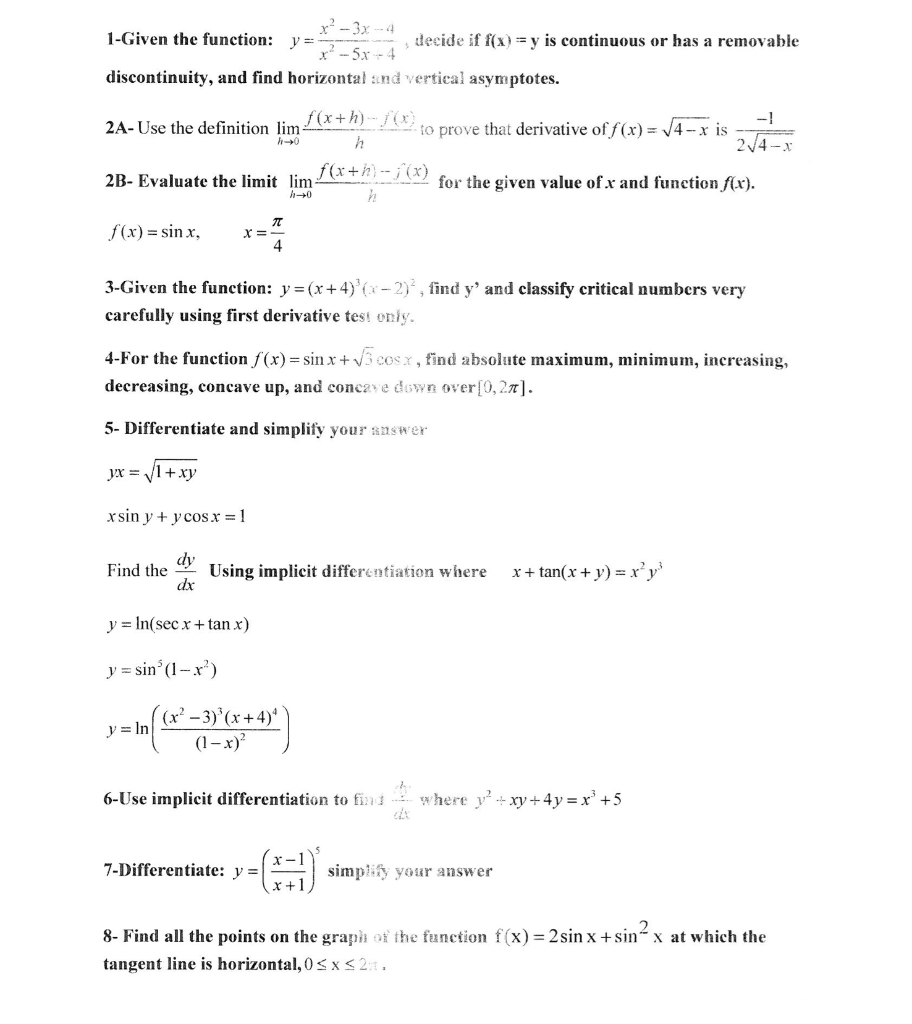r- 3X -4 1-Given the function: y=-- decide if f(x) = y is continuous or has a removable x? -5x -4 discontinuity, and find horizontal and vertical asymptotes. 2A-Use the definition lim 1(x+h)- / (8) to prove that derivative of f(x) = 14 - x is 24-x f(x+h)--; (x) 2B- Evaluate the limit lim for the given value of x and function f(x). 7 f(x) = sinx, X= 4 3-Given the function: y = (x+4)*(x-2), find y' and classify critical...

• ### Find the derivative of each one. a. y = (tan(x2 + 1))4 + 5 In Vx b. с. У-(sin x)cos x a. y = (tan(x2 + 1))4 + 5 In Vx b. с. У-(sin x)cos x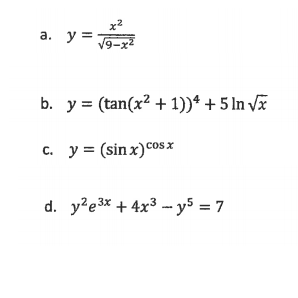Find the derivative of each one. a. y = (tan(x2 + 1))4 + 5 In Vx b. с. У-(sin x)cos x a. y = (tan(x2 + 1))4 + 5 In Vx b. с. У-(sin x)cos x

Free Homework App